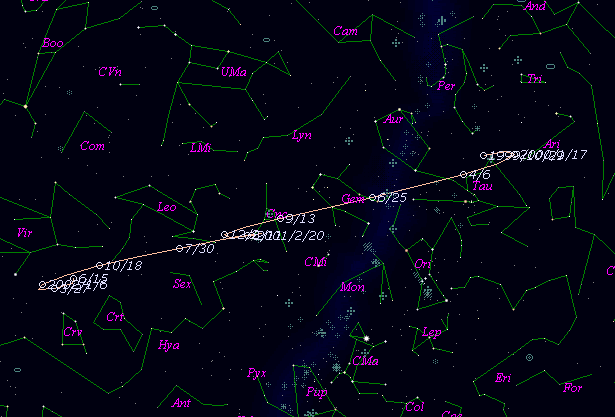\$B%+%?%j%JWB@1(B

P/1999 XN120 ( Catalina )\$B%W%m%U%#!<%k(B

 \$BH/8+F|(B 1999\$BG/(B12\$B7n(B5\$BF|(B \$BH/8+8wEY(B 16.9\$BEy(B \$BH/8+ Catalina Sky Survey\$B50F;MWAG(B

The following improved orbital elements by Kenji Muraoka, are
from 66 observations 1999 Nov. 3 to 2000 Mar. 18, perturbations
by 9 Planets, Moon and 5 minor planets were taken into account.
The mean residual is +/- 0.61 arc seconds.

Epoch  =  2000 May  16.0  TT       JDT = 2451680.5
T  =  2000 May   1.54310       +/- 0.07693 (m.e.) TT
Peri. =  161.82444                +/- 0.02698
Node  =  285.45532                +/- 0.03437   (2000.0)
Incl. =    5.03075                +/- 0.00732
q  =    3.2862679              +/- 0.0001965 AU
e  =    0.2131807              +/- 0.0000385
a  =    4.1766489              +/- 0.0001436 AU
n  =    0.11546813             +/- 0.00000595
P  =    8.536                  +/- 0.0004402  years
(+/- 0.16 day)\$B@1?^(B\$B8wEYJQ2=(B

m1 = 5.5 + 5 log\$B&\$(B + 20 log r\$B50F;MWAG\$OB<2,7r<#;a\$N7W;;\$K\$h\$k\$b\$N\$G\$9!#(B \$B@1?^\$O(B StellaNavigator Ver.2.0 for Windows (\$B%"%9%H%m%"!<%D(B \$BJTCx(B / \$B%"%9%-!<=PHG6I4)(B) \$B\$G:n@.\$7\$?\$b\$N\$G\$9!#(B \$B8wEY%0%i%U\$O(BComet for Windows\$B\$G:n@.\$7\$?\$b\$N\$G\$9!#(B